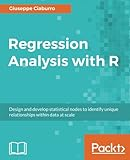# Regression Analysis with RRegression Analysis with R: Design and develop statistical nodes to identify unique relationships within data at scale

By 作者: Giuseppe Ciaburro

ISBN-10 书号: 178862730X

ISBN-13 书号: 9781788627306
Release Finelybook 出版日期: 2018-01-31
pages 页数: 422

\$39.99

Book Description to Finelybook sorting

Regression analysis is a statistical process which enables prediction of relationships between variables. The predictions are based on the casual effect of one variable upon another. Regression techniques for modeling and analyzing are employed on large set of data in order to reveal hidden relationship among the variables.
This book will give you a rundown explaining what regression analysis is, explaining you the process from scratch. The first few chapters give an understanding of what the different types of learning are – supervised and unsupervised, how these learnings differ from each other. We then move to covering the supervised learning in details covering the various aspects of regression analysis. The outline of chapters are arranged in a way that gives a feel of all the steps covered in a data science process – loading the training dataset, handling missing values, EDA on the dataset, transformations and feature engineering, model building, assessing the model fitting and performance, and finally making predictions on unseen datasets. Each chapter starts with explaining the theoretical concepts and once the reader gets comfortable with the theory, we move to the practical examples to support the understanding. The practical examples are illustrated using R code including the different packages in R such as R Stats, Caret and so on. Each chapter is a mix of theory and practical examples.
By the end of this book you will know all the concepts and pain-points related to regression analysis, and you will be able to implement your learning in your projects.

Contents
1: GETTING STARTED WITH REGRESSION
2: BASIC CONCEPTS – SIMPLE LINEAR REGRESSION
3: MORE THAN JUST ONE PREDICTOR – MLR
4: WHEN THE RESPONSE FALLS INTO TWO CATEGORIES – LOGISTIC REGRESSION
5: DATA PREPARATION USING R TOOLS
6: AVOIDING OVERFITTING PROBLEMS – ACHIEVING GENERALIZATION
7: GOING FURTHER WITH REGRESSION MODELS
8: BEYOND LINEARITY – WHEN CURVING IS MUCH BETTER
9: REGRESSION ANALYSIS IN PRACTICE
What You Will Learn
Get started with the journey of data science using Simple linear regression
Deal with interaction, collinearity and other problems using multiple linear regression
Understand diagnostics and what to do if the assumptions fail with proper analysis
Load your dataset, treat missing values, and plot relationships with exploratory data analysis
Develop a perfect model keeping overfitting, under-fitting, and cross-validation into consideration
Deal with classification problems by applying Logistic regression
Explore other regression techniques – Decision trees, Bagging, and Boosting techniques
Learn by getting it all in action with the help of a real world case study.
Authors
Giuseppe Ciaburro
Giuseppe Ciaburro holds a PhD in environmental technical physics and two master’s degrees. His research is on machine learning applications in the study of urban sound environments. He works at Built Environment Control Laboratory, Università degli Studi della Campania Luigi Vanvitelli (Italy). He has over 15 years’ experience in programming Python, R, and MATLAB, first in the field of combustion, and then in acoustics and noise control. He has several publications to his credit.

### 觉得文章有用就赞赏一下文章作者

#### 支付宝扫一扫打赏#### 微信扫一扫打赏• QQ咨询
• 回顶